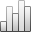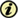Acta Stereologica

0351-580XMore Statisticssince 05 December 2013 :
View(s): 264 (1 ULiège)
Peter Edgar Rose

### The estimation of errors in evaluating sphere size distribution due to variations in section thickness

Article#### Abstract

A simple expression is presented for the estimation of the errors in unfolding sphere size distributions and population densities incurred by deviations in the actual section thickness from its putative value. The error factor in determining population density for given actual and putative section thicknesses (Rose,1982) is expressed as a scalar multiple of a matrix for unfolding population densities and may readily be used in conjunction with the tables of these matrices previously published (Rose, 1980). It is shown that if the probability distribution of section thicknesses in the sampling fields is known, an estimate of the gross error in estimating population density incurred by variations in section thickness in the sampling fields may be made. It is further shown that in general the overall error in estimating population density is zero only if the probability distribution of section thicknesses is symmetrical about the putative value.